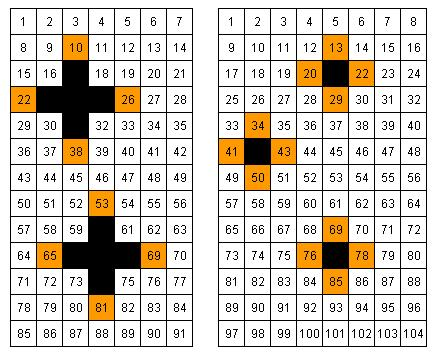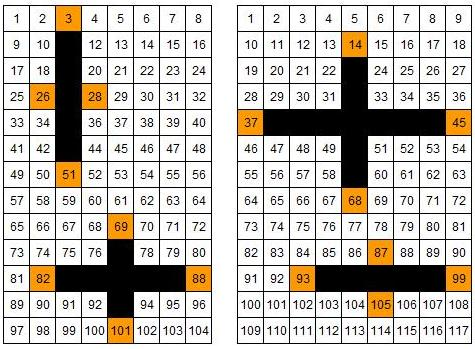#### You may also like### Summing Consecutive Numbers

15 = 7 + 8 and 10 = 1 + 2 + 3 + 4. Can you say which numbers can be expressed as the sum of two or more consecutive integers?### Always the Same

Arrange the numbers 1 to 16 into a 4 by 4 array. Choose a number. Cross out the numbers on the same row and column. Repeat this process. Add up you four numbers. Why do they always add up to 34?### Fibs

The well known Fibonacci sequence is 1 ,1, 2, 3, 5, 8, 13, 21.... How many Fibonacci sequences can you find containing the number 196 as one of the terms?

# Crossed Ends

##### Age 11 to 14Challenge LevelThese crosses can be drawn on number grids of various sizes.

Add opposite pairs of orange numbers
(i.e. north + south, east + west).

Notice anything?

Try a few more.Now try adding opposite pairs on crosses with just two lines of symmetry, like these:

What do you notice?

Experiment with grids of various sizes.

You may find these printable sheets useful:
Number Grids - width 3, 4, 5 and 6
Number Grids - width 7 and 8
Number Grids - width 9 and 10

What happens if you add the orange squares in adjacent pairs? (try N + W, S + E )

Can you predict in advance how the totals will relate to each other?
What does it depend on?
Is it the same if you added them the other way round? (i.e. N + E, S + W)